# Extract the skin from a mesh#

Extracting the skin of a mesh to reduce the amount of data to operate on can be useful for specific results and for performance.

Note

```# Import necessary modules
from ansys.dpf import core as dpf
from ansys.dpf.core import examples
from ansys.dpf.core import operators as ops

```

Create a model object to establish a connection with an example result file and then extract:

```model = dpf.Model(examples.download_multi_stage_cyclic_result())
print(model)
```
```DPF Model
------------------------------
Modal analysis
Unit system: MKS: m, kg, N, s, V, A, degC
Physics Type: Mechanical
Available results:
-  displacement: Nodal Displacement
-  stress: ElementalNodal Stress
-  elastic_strain: ElementalNodal Strain
-  structural_temperature: ElementalNodal Temperature
------------------------------
DPF  Meshed Region:
3595 nodes
1557 elements
Unit: m
With solid (3D) elements
------------------------------
DPF  Time/Freq Support:
Number of sets: 6
Cumulative     Frequency (Hz) LoadStep       Substep        Harmonic index
1              188.385357     1              1              0.000000
2              325.126418     1              2              0.000000
3              595.320548     1              3              0.000000
4              638.189511     1              4              0.000000
5              775.669703     1              5              0.000000
6              928.278013     1              6              0.000000
```

# Create the workflow#

Maximum principal stress usually occurs on the skin of the model. Computing results only on this skin reduces the data size.

```# Create a simple workflow computing the principal stress on the skin
# of the model.

skin_mesh = skin_op.outputs.mesh()
```

Plot the mesh skin:

```skin_mesh.plot()
```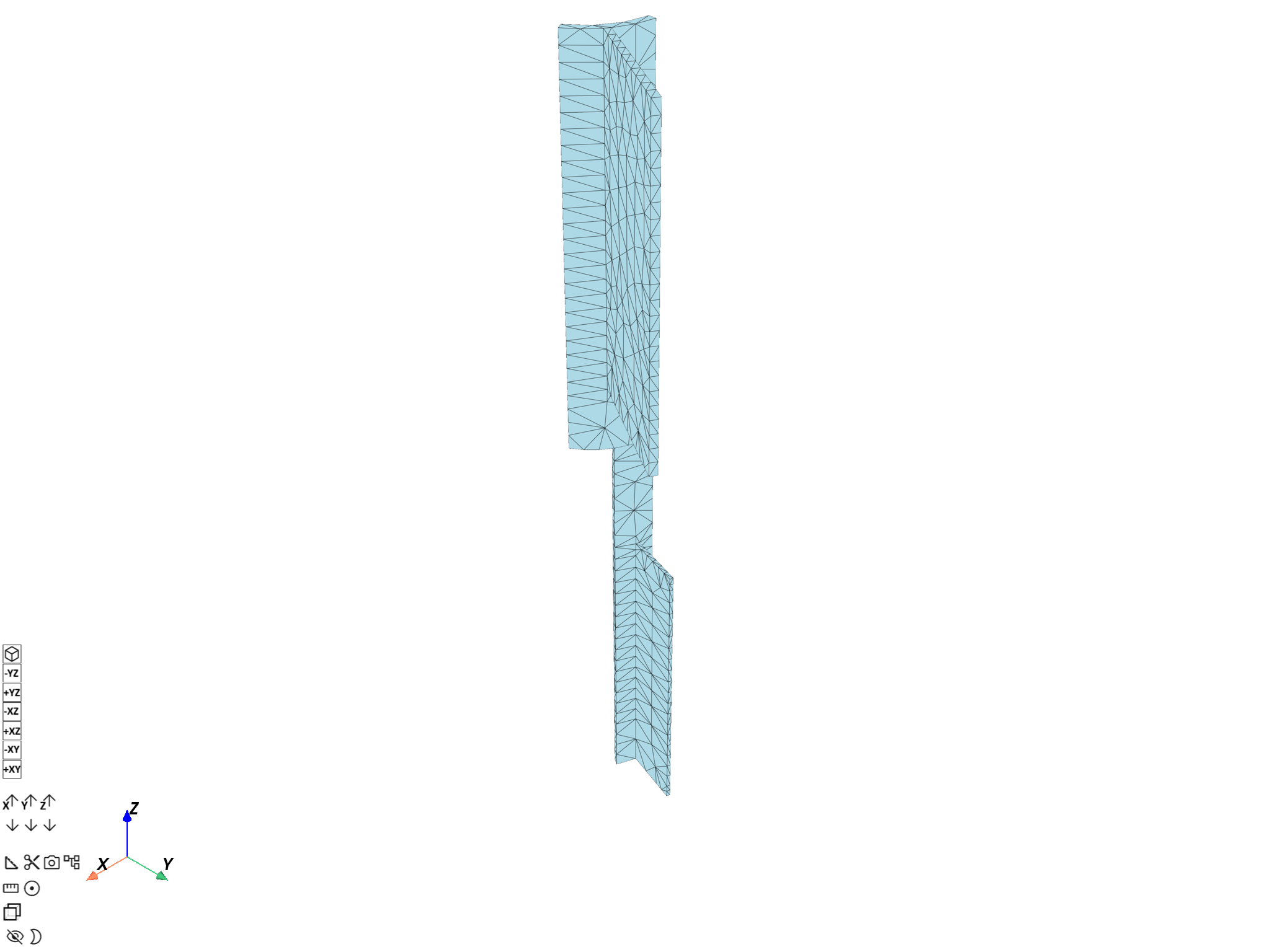Compute the stress principal invariants on the skin nodes only:

```stress_op = ops.result.stress(data_sources=model.metadata.data_sources)
stress_op.inputs.requested_location.connect(dpf.locations.nodal)
stress_op.inputs.mesh_scoping.connect(skin_op.outputs.nodes_mesh_scoping)

principal_op = ops.invariant.principal_invariants_fc(stress_op)
principal_stress_1 = principal_op.outputs.fields_eig_1()
principal_stress_2 = principal_op.outputs.fields_eig_2()
principal_stress_3 = principal_op.outputs.fields_eig_3()
```

# Manipulate data locally#

This example goes over the fields, keeping the largest invariant value by node if the averaged value of invariants is large enough. Exploring data allows you to customize it to meet your needs.

```node_scoping_ids = principal_stress_1.scoping.ids
threshold = 300000.0

field_to_keep = dpf.fields_factory.create_scalar_field(
len(node_scoping_ids), location=dpf.locations.nodal
)

with field_to_keep.as_local_field() as f:
with principal_stress_1.as_local_field() as s1:
with principal_stress_2.as_local_field() as s2:
with principal_stress_3.as_local_field() as s3:
for i, id in enumerate(node_scoping_ids):
d1 = abs(s1.get_entity_data_by_id(id))
d2 = abs(s2.get_entity_data_by_id(id))
d3 = abs(s3.get_entity_data_by_id(id))
if (d1 + d2 + d3) / 3.0 > threshold:
d = max(d1, d2, d3)
f.append(d, id)
```

# Plot result field#

Plot the result field on the skin mesh:

```skin_mesh.plot(field_to_keep)
```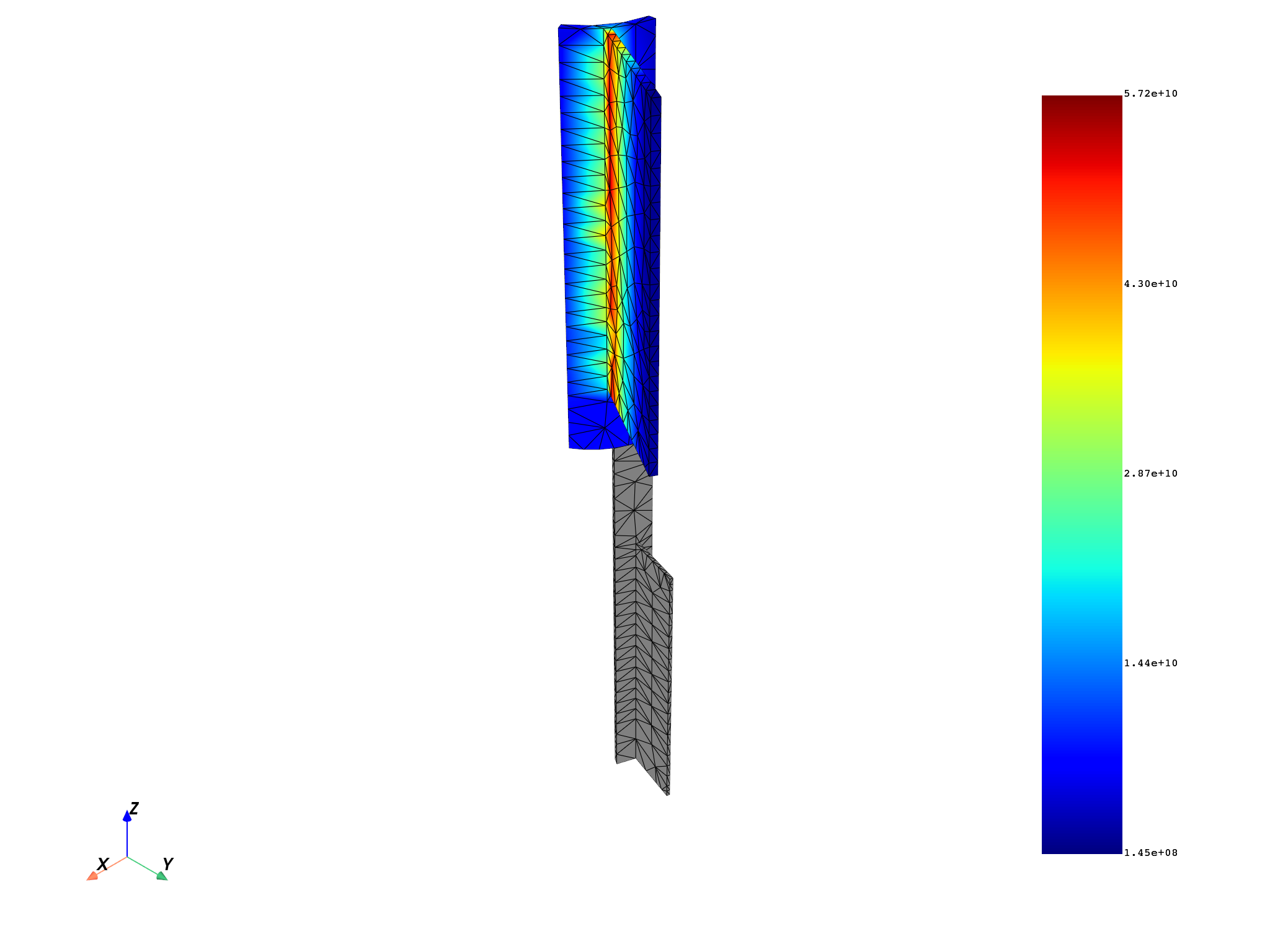# Plot initial invariants#

Plot the initial invariants on the skin mesh:

```skin_mesh.plot(principal_stress_1)
skin_mesh.plot(principal_stress_2)
skin_mesh.plot(principal_stress_3)
```
•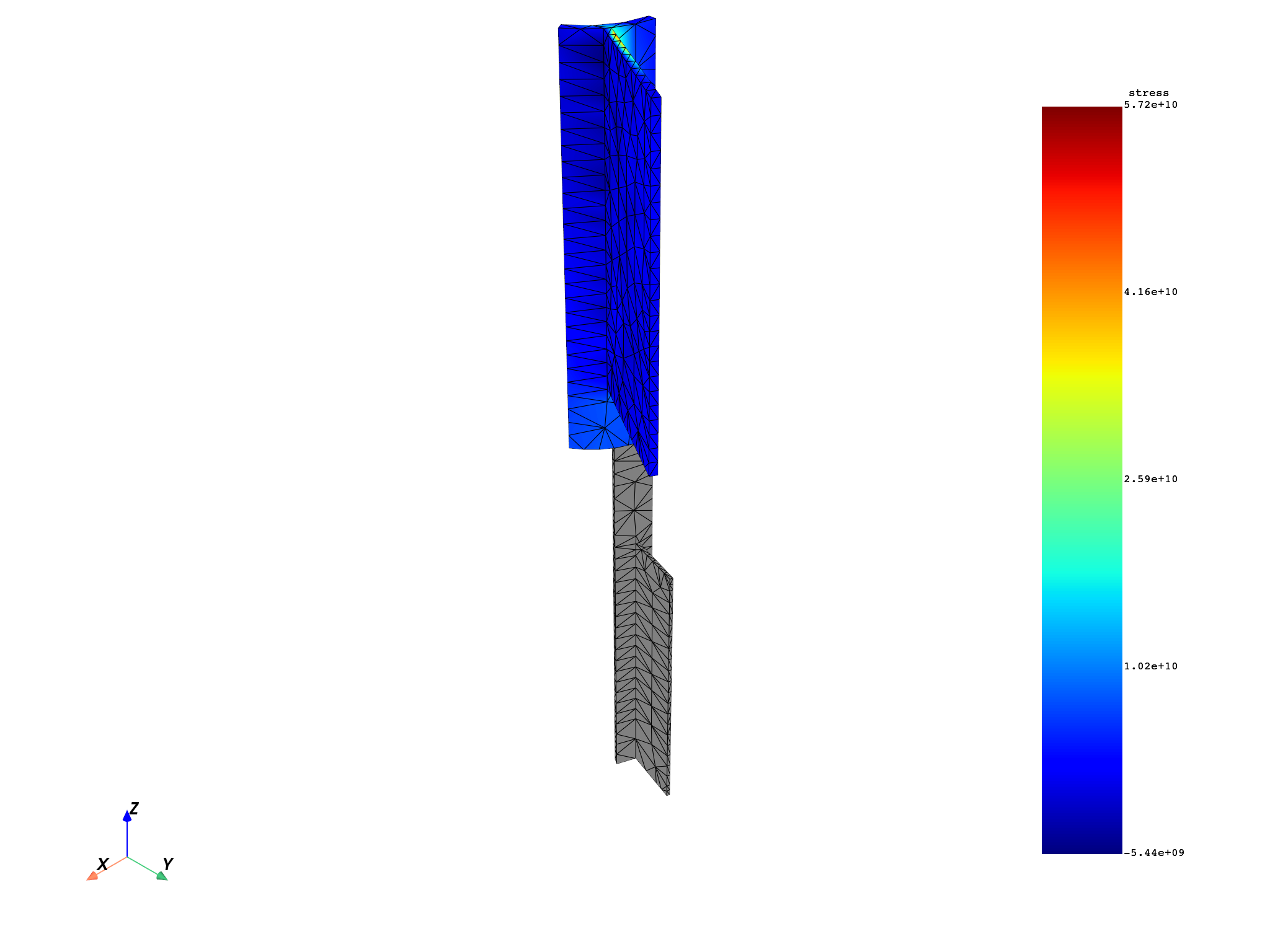•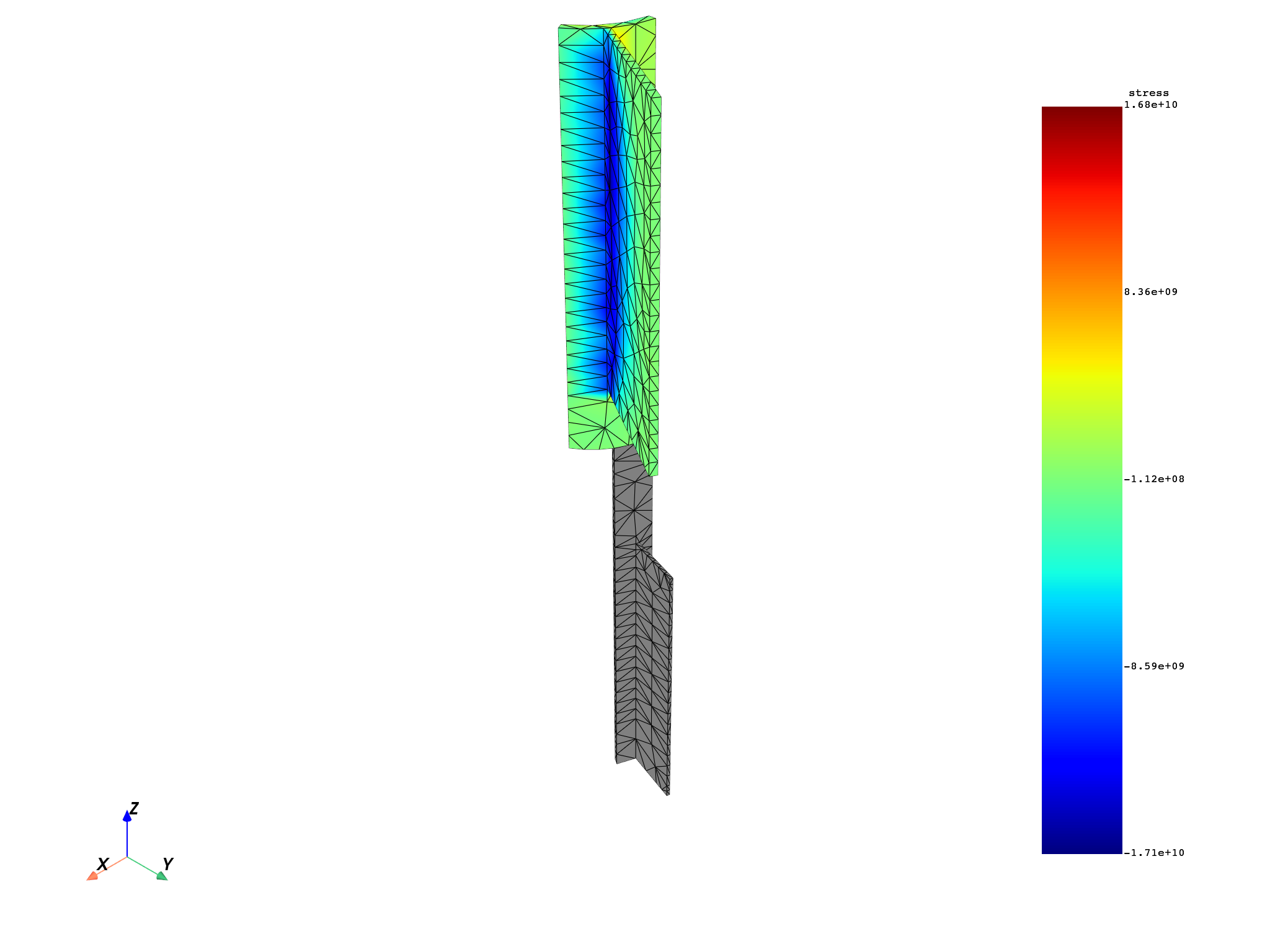•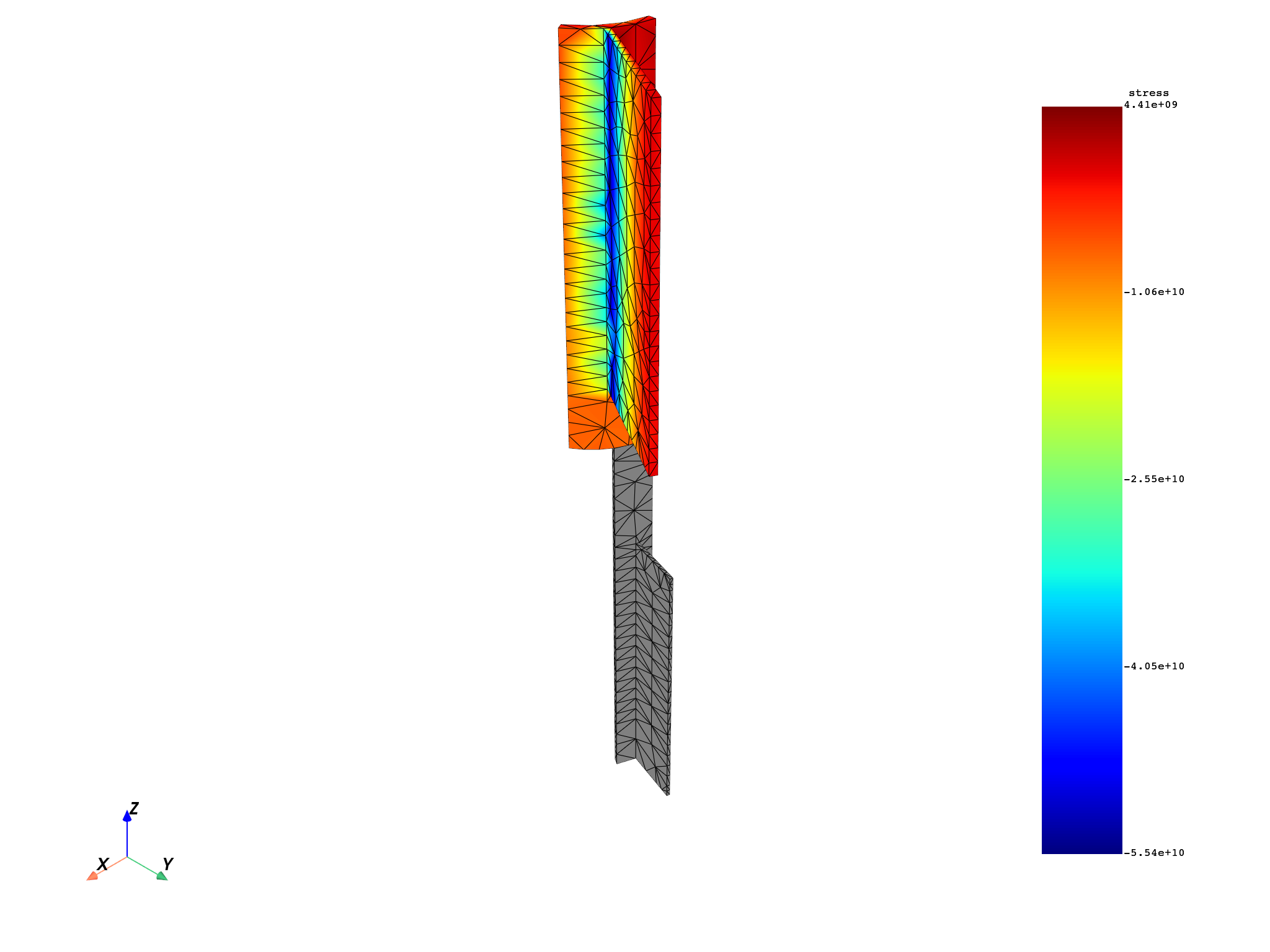Total running time of the script: ( 0 minutes 5.566 seconds)

Gallery generated by Sphinx-Gallery